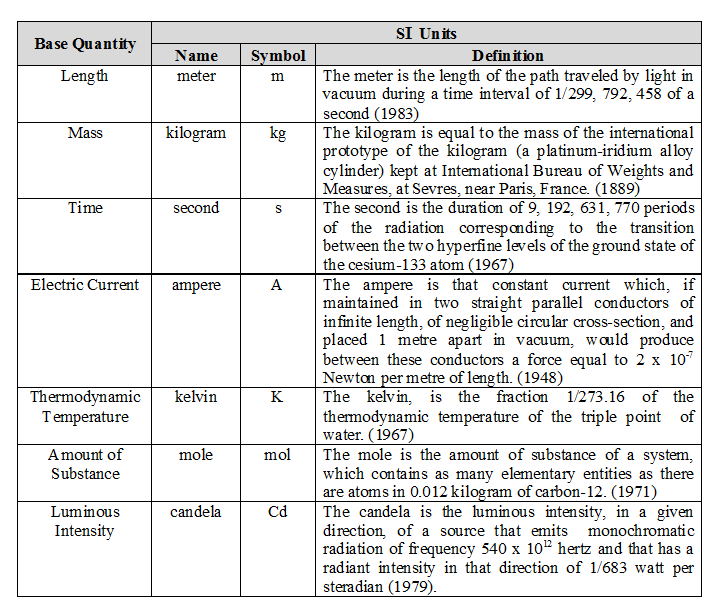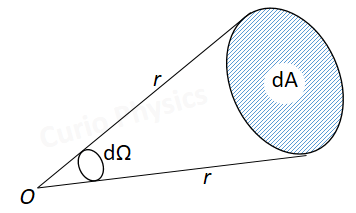# System of Units

##### System of Units :-

A system of units is the complete set of units, both fundamental and derived, for all kinds of physical quantities. The common system of units which is used in mechanics are given below :-

(a) CGS or Gaussian system
In this system the fundamental quantities are length, mass and time and their respective units are centimeter (cm), gram (g) and second (s).

(b) FPS or British Engineering system

In this system length, mass and time are taken as fundamental quantities and their base units are foot (ft), pound (lb.) and second (s) respectively.

(c) MKS system
In this system also the fundamental quantities are length, mass and time but their fundamental units are meter (m), kilogram (kg) and second (s) respectively.

(d) International system (SI) of units :
This system is modification over the MKS system and so it is also known as Rationalized MKS system. Besides the three base units of MKS system four fundamental and two supplementary units are also included in this system.
The SI system is at present widely used throughout the world.Supplementary units

In SI, two supplementary units are also defined viz. (i) radian (rad) for plane angle and (ii) steradian (sr) for solid angle.
(i) radian : 1 radian is the angle subtended at the Centre of a circle by an arc equal in length to the radius of the circle.$\displaystyle d\theta =\frac{ds}{r}$

If ds = r then dθ = 1 Radian

1 Radian = (180/π) degrees = 57.3 degrees

(ii) steradian : 1 steradian is the solid angle subtended at the Centre of a sphere, by that surface of the sphere which is equal in area to the square of the radius of the sphere.Solid angle(dΩ) = dA/r2

If dA = r2 then dΩ = 1 steradian

The solid angle subtended at the center by the total surface area:-

Ω = 4πr2/r2 = 4π steradian

Practical units :

Due to the fixed sizes of SI units, some practical units are also defined for both fundamental and derived quantities. e.g. light year (ly) is a practical unit of astronomical distance (a fundamental quantity) and horse power (hp) is a practical unit of power (a derived quantity). Practical units may or may not belong to a particular system of units but can be expressed in any system of units. e.g. 1 mile = 1.6 km = 1.6 × 103 m =1.6 × 105 cm.

Improper units :

These are the units which are not of the same nature as that of the physical quantities for which they are used. e.g. kg-wt. is an improper unit of weight. Here kg is a unit of mass but it is used to measure the weight (force).

Next Topic :-    Dimensions